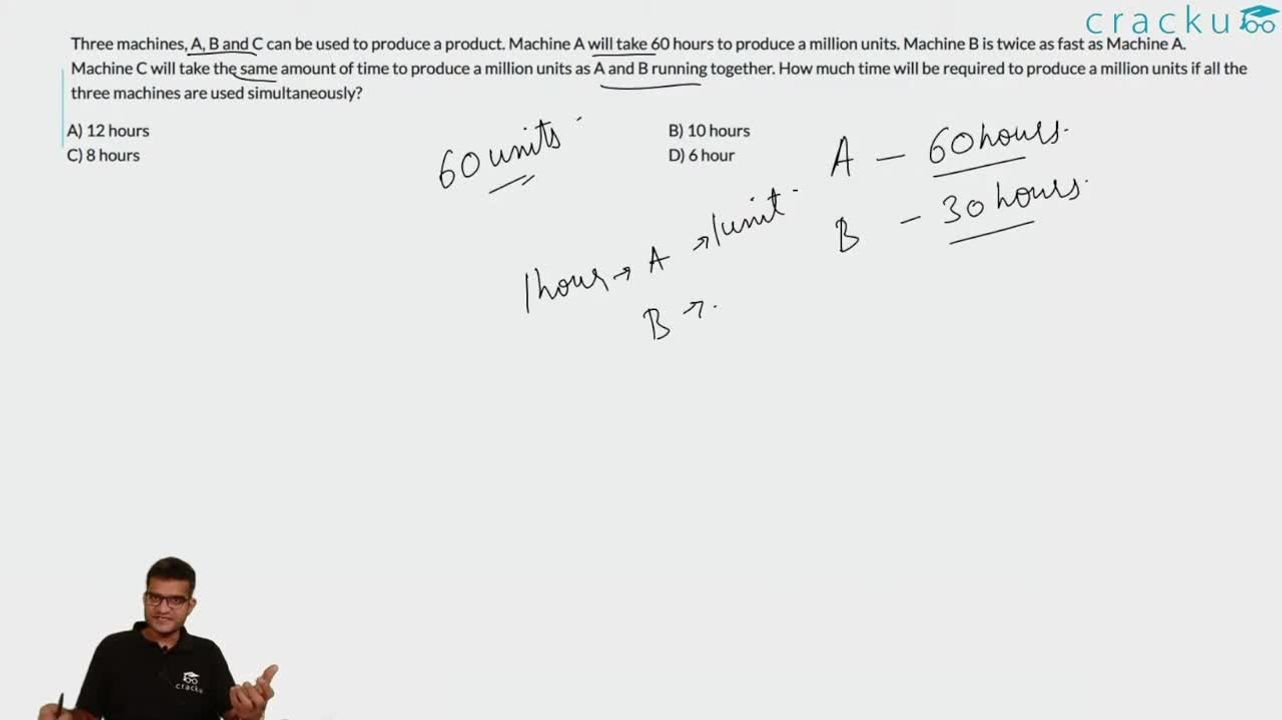Question 29

# Three machines, A, B and C can be used to produce a product. Machine A will take 60 hours to produce a million units. Machine B is twice as fast as Machine A. Machine C will take the same amount of time to produce a million units as A and B running together. How much time will be required to produce a million units if all the three machines are used simultaneously?

Solution

As machine B's efficiency is twice as of A's, Hence, it will complete its work in 30 hours.
And C's efficiency is putting A and B together i.e. = 20 hours $$( (\frac{1}{60} + \frac{1}{30})^{-1})$$
Now if all three work together, then it will be completed in x (say) days.

$$\frac{1}{x} = \frac{1}{20} + \frac{1}{30} + \frac{1}{60}$$

or x = 10 hours

### View Video Solution